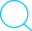# 对TTL反相器的一些原理的整理

## 知识总结

Posted by Kingtous on July 3, 2019

## 对TTL反相器的一些原理的整理### 定性分析

• 当输入是低电平(0.2V)

$T_1$的基极电流$i_{B1}$流入发射极，也就是说从反相器的输入端流出，所以$i_{B2}$约等于0，所以$T_2$截止，$T_5$也截止，$T_4$和$D_2$将导通，输出高电平

• 当输入是高电平(3.6V)

$T_1$倒置，也就是说发射极和集电极颠倒，$i_{B1}$流入$T_2$基极，使得$T_2$饱和导通，$T_5$亦然，$T_4$和$D_2$截止，输出低电平

### 定量分析

• 当输入是高电平(3.6V)时

如不考虑$T_2$，$T_5$的存在时，$T_1$的基极电位将会是4.3V，但由于有$T_2$，$T_5$的存在，$T_1$的基极电位会被嵌在2.1V，这时$T_2$，$T_5$都是导通的，而且还是饱和导通的状态（偏置电流较大的原因吧，导致饱和导通），故$V_{c2}$=0.7+0.2=0.9V(0.2V为饱和压降，即集电极会比发射极高0.2V)，0.9V的电压是不足以使$T_4$导通的（至少要2x0.7V=1.4V），因此$T_4$截止，Y的输出电压就是0.2V了($T_5$的发射极电位为0，集电极则为0.2V，还是因为饱和压降)，是低电平。• 当输入是低电平(0.2V)时

发射结导通，基极电流流入发射极，$v_{B1}$($T_1$的基级电位)等于输入的低电平+0.7V=0.9V，而导通T2和T5分别需要0.7+0.7=1.4V和0.7+0.7+0.7(T1的集电结+T2的发射结+T5的发射结)=2.1V，所以$T_2$和$T_5$截止。所以$T_2$相当于一个无穷大的电阻，压降无穷大，所以$T_4$的基极电位可看作5V，在经过发射结和$D_2$时分别下降0.7V，故$v_0$为5-0.7-0.7=3.6V，输出高电平。

### 参考

• 发烧友